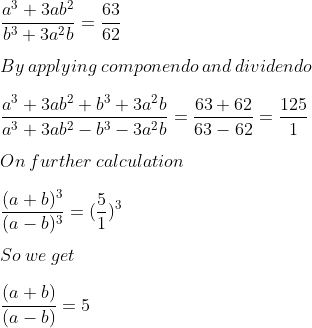Guru

# Given that a³+3ab²/(b³+3a²b) =63/62. Using componendo and dividendo find a: b.

• 0

exam oriented an important question from ML aggarwal, class 10th, chapter 7, ratio and proportion, Avichal publication

This question has been asked in previous year question paper 2009

In this question we have been given an equation and by using componendo dividendo, we have to find a:b

Quesn 16, 7.3

Share

1. Solution:

It is given thatBy cross multiplication

a + b = 5a – 5b

We can write it as

5a – a – 5b – b = 0

4a – 6b = 0

4a = 6b

We get.

a/b = 6/4

a/b = 3/2

∴ a: b = 3: 2

• 0